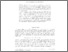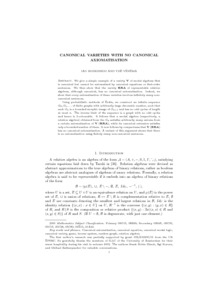# PP-2003-13: Canonical varieties with no canonical axiomatisation

PP-2003-13: Hodkinson, Ian and Venema, Yde (2003) Canonical varieties with no canonical axiomatisation. [Report]Preview Text (Full Text) PP-2003-13.text.pdf Download (352kB) | PreviewText (Abstract) PP-2003-13.abstract.txt Download (1kB)

## Abstract

We give a simple example of a variety V of modal algebras that is
canonical but cannot be axiomatised by canonical equations or
first-order sentences. We then show that the variety RRA of
representable relation algebras, although canonical, has no
canonical axiomatisation. Indeed, we show that every axiomatisation
of these varieties involves infinitely many non-canonical sentences.

Using probabilistic methods of Erdos, we construct an infinite
sequence G_0,G_1,... of finite graphs with arbitrarily large chromatic
number, such that each G_n is a bounded morphic image of G_{n+1} and
has no odd cycles of length at most n. The inverse limit of the
sequence is a graph with no odd cycles and hence is 2-colourable.
It follows that a modal algebra (respectively, a relation algebra)
obtained from the G_n satisfies arbitrarily many axioms from a
certain axiomatisation of V (RRA), while its canonical extension
satisfies only a bounded number of them. It now follows by
compactness that V (RRA) has no canonical axiomatisation.
A variant of this argument shows that there is no axiomatisation
using finitely many non-canonical sentences.

Item Type: Report PP-2003-13 Prepublication (PP) Series 2003 Canonical axiomatisation, canonical equation, canonical modal logic, canonical variety, game, inverse system, random graph, relation algebra Logic 12 Oct 2016 14:36 12 Oct 2016 14:36 https://eprints.illc.uva.nl/id/eprint/97View Item